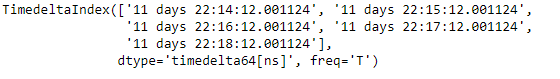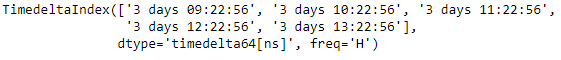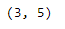# Python | Pandas TimedeltaIndex.slice_locs()

Python is a great language for doing data analysis, primarily because of the fantastic ecosystem of data-centric python packages. Pandas is one of those packages and makes importing and analyzing data much easier.

Pandas` TimedeltaIndex.slice_locs()` function compute the slice location for input labels and step for an ordered TimedeltaIndex object. The function assumes that the data is sorted.

Syntax : TimedeltaIndex.slice_locs(start=None, end=None, step=None, kind=None)

Parameters :
start : (label, default None) If None, defaults to the beginning
end : (label, default None) If None, defaults to the end
step : (int, defaults None) If None, defaults to 1
kind : {‘ix’, ‘loc’, ‘getitem’} or None

Return : start, end : int

Example #1: Use `TimedeltaIndex.slice_locs()` function to compute the slice location for the passed label in the given TimedeltaIndex object.

 `# importing pandas as pd ` `import` `pandas as pd ` ` `  `# Create the TimedeltaIndex object ` `tidx ``=` `pd.TimedeltaIndex(start ``=``'11 days 22:14:12.001124'``, periods ``=` `5``, freq ``=``'T'``) ` ` `  `# Print the TimedeltaIndex object ` `print``(tidx) `

Output :Now we will use the `TimedeltaIndex.slice_locs()` function to find the slice location value for the passed labels.

 `# # find the slice location for the passed label ` `tidx.slice_locs(``'11 days 22:15:20.001124'``) `

Output :As we can see in the output, the `TimedeltaIndex.slice_locs()` function has returned the start position as well as the end position of the slice in the tidx object.

Example #2: Use `TimedeltaIndex.slice_locs()` function to compute the slice location for the passed label in the given TimedeltaIndex object.

 `# importing pandas as pd ` `import` `pandas as pd ` ` `  `# Create the TimedeltaIndex object ` `tidx ``=` `pd.TimedeltaIndex(start ``=``'03 days 09:22:56'``, periods ``=` `5``, freq ``=``'H'``) ` ` `  `# Print the TimedeltaIndex object ` `print``(tidx) `

Output :Now we will use the `TimedeltaIndex.slice_locs()` function to find the slice location value for the passed labels.

 `# find the slice location for the passed label ` `tidx.slice_locs(``'3 days 12:20:56'``) `

Output :As we can see in the output, the `TimedeltaIndex.slice_locs` function has returned the start position as well as the end position of the slice in the tidx object.

My Personal Notes arrow_drop_upCheck out this Author's contributed articles.

If you like GeeksforGeeks and would like to contribute, you can also write an article using contribute.geeksforgeeks.org or mail your article to contribute@geeksforgeeks.org. See your article appearing on the GeeksforGeeks main page and help other Geeks.

Please Improve this article if you find anything incorrect by clicking on the "Improve Article" button below.

Article Tags :

Be the First to upvote.

Please write to us at contribute@geeksforgeeks.org to report any issue with the above content.